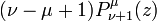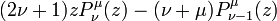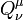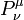# User:Tohline/Math/EQ Toroidal04

(Difference between revisions)$~(\nu - \mu + 1)P^\mu_{\nu + 1} (z)$$~=$$~ (2\nu + 1)z P_\nu^\mu(z) - (\nu + \mu)P^\mu_{\nu-1}(z)$ Abramowitz & Stegun (1995), p. 334, eq. (8.5.3)
 NOTE:$~Q_\nu^\mu$, as well as$~P_\nu^\mu$, satisfies this same recurrence relation.